# L Section in Four Terminal Network

## L Section in Four Terminal Network:

L Section in Four Terminal Network is very much similar to half section consisting series and shunt arm impedances Z1 and Z2 respectively as shown in the Fig. 8.28. The ladder network can be analyzed into a series of L sections rather than T and π sections. The main application of L section is in matching of impedances between two sections.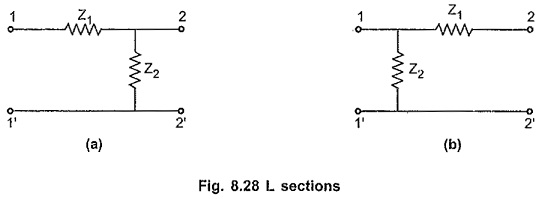### Interative Impedance (Z′0):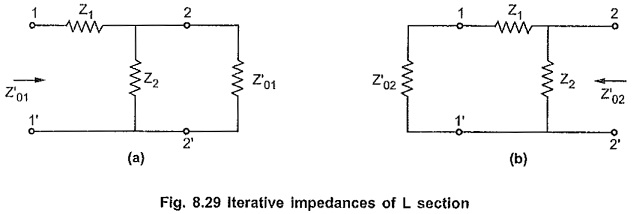Consider Fig. 8.29 (a),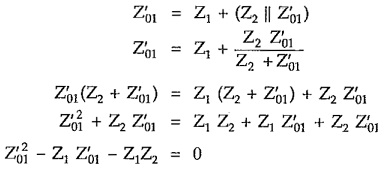Finding roots, we can write,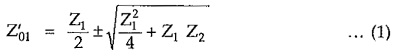Consider Fig. 8.29 (b),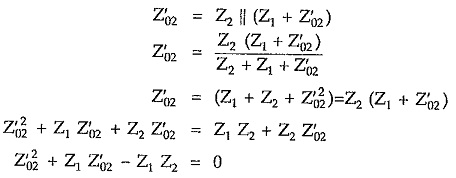Finding roots, we can write,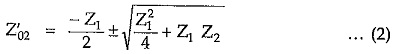### Image Impedances (Zi):

Consider Fig. 8.29 (a)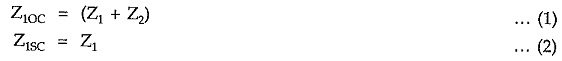Thus, image impedance Zi1 at port 1 is given by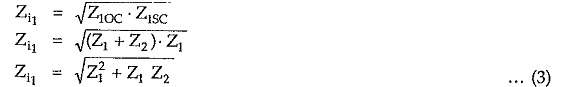Similarly,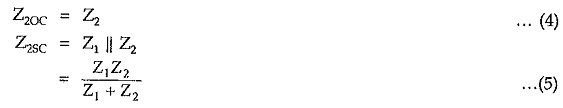Thus, image impedance Zi2 at port 2 is given by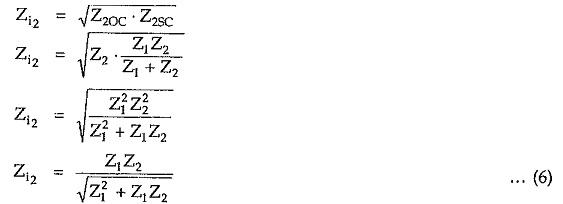Updated: November 9, 2019 — 6:08 pm